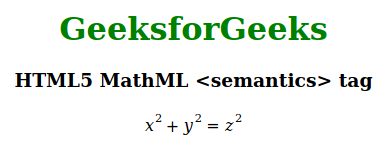# HTML5 | MathML <semantics> Tag

• Last Updated : 30 Dec, 2019

The HTML5 MathML <semantics> tag is an inbuilt element in HTML 5. It is used to markup the mathematics there are two possible ways to markup mathematics.

• Presentation MathML is used to control the layout of any mathematical equations.
• Content MathML is designed to encode the semantic mathematical meaning and make expressions understandable to computer algebra systems.

This tag act as container elements that should contain child elements. There are tow more tag that performs with this tag those are <annotation> tag and the <annotation-xml>. This tag The <annotation> element is the container of those element and containing semantic information in a non-XML format, whereas the <annotation-xml> element contains content in an XML format, like Content MathML or OpenMath.

Attention reader! Don’t stop learning now. Get hold of all the important HTML concepts with the Web Design for Beginners | HTML  course.

Syntax:

`<semantics> child elements </semantics>`

Attributes: This tag accepts below mentioned attributes and have to use those attributes on <annotation> and <annotation-xml>.

• definitionURL: This attribute holds the location of the annotation symbol.
• encoding: This attribute used to encode the semantic information in the annotation.
• cd: This attribute is used to holds the annotation symbols.
• name: This attribute holds the name of the annotation key symbol.
• src: This attribute holds the location of an external source for semantic information.

Below example illustrates the <semantics> tag in HTML 5:

 ```<``html``>`` ` `<``head``>``    ``<``title``>HTML 5 MathML <``semantics``> tag```` ` `<``body``>``    ``<``center``>``        ``<``h1` `style``=``"color:green"``> ``            ``GeeksforGeeks ``        ```` ` `        ``<``h3``>HTML5 MathML <``semantics``> tag`` ` `        ``<``math``>``            ``<``semantics``>`` ` `                ````                ``<``mrow``>``                    ``<``msup``>``                        ``<``mi``>x``                        ``<``mn``>2``                    ````                    ``<``mo``>+``                    ``<``msup``>``                        ``<``mi``>y``                        ``<``mn``>2``                    ````                    ``<``mo``>=``                    ``<``msup``>``                        ``<``mi``>z``                        ``<``mn``>2``                    ````                ```` ` `                ````                ``<``annotation-xml` `encoding``=``"MathML-Content"``>``                    ``<``apply``>``                        ``<``plus``/>``                        ``<``apply``>``                            ``<``power``/>``                            ``<``ci``>x``                            ``<``cn` `type``=``"integer"``>2``                        ````                        ``<``apply``>``                            ``<``power``/>``                            ``<``ci``>y``                            ``<``cn` `type``=``"integer"``>2``                        ````                        ``<``equal``/>``                        ``<``apply``>``                            ``<``power``/>``                            ``<``ci``>z``                            ``<``cn` `type``=``"integer"``>2``                        ````                    ````                ```` ` `                ````                ``<``annotation` `encoding``=``"image/png"` `src``=``"https://media.geeksforgeeks.org/wp-content/uploads/20191226151654/762.png"` `/>`` ` `                ````                ``<``annotation` `encoding``=``"application/x-tex"``>``                    ``x^{2} + y^{2} = z^{2}``                ```` ` `            ````        ````    ``````                    `

Output:Supported Browsers: The browsers supported by HTML 5 MathML <semantics> tag are listed below:

• Firefox

My Personal Notes arrow_drop_up